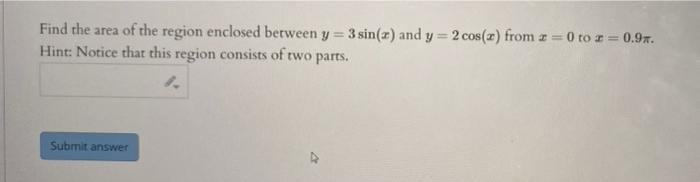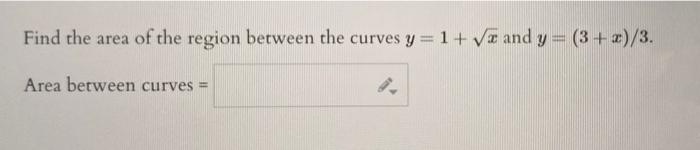# (Solved): Hello, can you please help me with these questions? Thank you so much! Find the area of the region e ...Find the area of the region enclosed between $$y=3 \sin (x)$$ and $$y=2 \cos (x)$$ from $$x=0$$ to $$x=0.9 \pi$$. Hint: Notice that this region consists of two parts. Find the area of the region between the curves $$y=1+\sqrt{x}$$ and $$y=(3+x) / 3$$.

We have an Answer from Expert